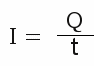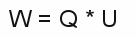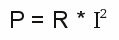# Current, resistance and power

## Terminology current

Electric current is synonymic with moving charge. As we have seen at the previous chapter, an electric field exerts a push or pull on a charge. If the charge is not fixed, the electric field causes it to move straight away, depending on the direction of the field and the sign of the charge. The stronger the electric field, the stronger the force and the faster they accelerate. The faster the charge(s) move, the higher the value of the current becomes. The number of charges being accelerated by the electric field influences the value of the electric current, too. The electric current can be defined as the number of moving elementary charges per time, or the amount of charge per time:

[3.6a]while the electric current is constant, we get
[3.6b]Where is:
I - Electric current, Q - charge, t - time

The symbol used for the electric current is I and the unit Symbol is A, meaning Ampere, named after the French physicist André Marie Ampère.

## Resistance

Until now we just investigated two interacting charged particles or just one single charge inside an electric field. According to its charge and the strength of the electric field, there is a resulting force. Like explained at the chapter about mechanics, a force accelerates objects. According to this, a charged particle becomes permanently faster while being inside an electric field, meaning the current increases. This statement is valid as long as there is no friction. Scientists take big efforts to eliminate those friction inside particle accelerators. The charged particles are kept inside evacuated tubes to avoid collisions with neutral Atoms (which are not or just weakly accelerated by electric fields). Those collisions slow down the accelerated particles again and again - the collisions correspond to the friction (air and rolling resistance) at the chapter mechanics. At the presence of friction, the charged particles reach a maximum speed inside the electric field and stop accelerating. The processes slowing down electrons while colliding with other particles (atoms, other electrons) are much more complex than the friction discussed at the chapter mechanics, but they also lead to a loss of directed movement in favor of nondirectional movement of the surrounding particles. That's simple-minded increasing temperature.
In practice, electrons are not moving in evacuated tubes, but inside metal wires. Metal fulfills an important condition, making it possible to move electrons inside an electric field: At metallic bondings there are some electrons being just weakly bond to their atomic cores. If all electrons would be fixed at their metallic nuclei, they could not be accelerated by an electric field. The mechanical analog is the fact that an object can't be moved as long as the attracting force is smaller than the static friction.
The disadvantage of metal wire is the fact that besides the needed free electrons there are many Atoms respectively atom nuclei, too. The electrons being accelerated by the electric field interact with those particles and the resulting friction is inevitable. The friction of moving electrons inside a wire is called electric resistance.

## Terminology electric resistance

At the previous chapter we saw that the strength of an electric field is given by the difference of potential between two points, given as voltage. Caused by friction (resistance) there is a maximum speed, electrons reach while being accelerated by the according field. The number of electrons moving inside a wire is limited, too and so there is a maximum value of electric current. By increasing the voltage (=the strength of the electric field), the maximum speed of the electrons and so the value of the electric current increases, too. Vice versa the electric current decreases while the voltage gets lower. While the temperature is constant, the correlation between voltage and current is linear inside a metallic wire. So the correlation between voltage, current and resistance is given by:

[3.7]Where is:
R - Resistance, I - current, U - voltage

This correlation was first discovered by the German physicist Georg Simon Ohm. In his honor it is called "Ohm's law". The symbol of the resistance is R and the unit is called Ohm. The unit symbol is the Greek letter Ω (Omega).

## Terminology Power

As mentioned before, voltage gives the work per charge done by an electric field. The electric current is defined as the moving charge per time. At the chapter about mechanics you can see that power is defined as work (or transformed energy ) per time. So we get:insertingandgives:
[3.8]Using formula [3.7] for the electric resistance, we get:oderWhere is:
W - Work, I - current, U - voltage, P - power, Q - charge, t - time, R - resistance

The symbol used is analog to mechanical power P, and the SI unit is the watt.